# Lavrent'ev theorem

(diff) ← Older revision | Latest revision (diff) | Newer revision → (diff)

Lavrent'ev's theorem in descriptive set theory: A topological mapping between two sets incan be extended to a homeomorphism between certain sets of type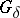containing them. A consequence of this theorem is that the Hausdorff type of a set is a topological invariant (see ).

Lavrent'ev's theorem in approximation theory gives a criterion for the possibility of uniform approximation: In order that a function, continuous on a compact set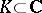, can be uniformly approximated onby polynomials it is necessary and sufficient thatis a compact set without interior points that does not partition the complex plane (see ).

Lavrent'ev's theorem in the theory of quasi-conformal mapping: Let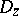andbe two simply-connected domains in the plane bounded by piecewise-smooth curves and letand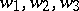be triples of positively enumerated points on their boundaries. Then for any strongly-elliptic system of equations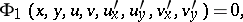with uniformly continuous partial derivatives of the functions that specify the equations of the characteristics, there is always a unique homeomorphic mapping ofonto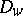that realizes a solution,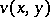of the system, and under which the given triples of boundary points correspond to each other.

For Lavrent'ev's theorem in mechanics (aerofoil theory, solitary wave, forms of dynamical loss of stability, flows, the theory of cumulative charge, directed detonation) see .

Theorems 1)–4) are due to M.A. Lavrent'ev.

How to Cite This Entry:
Lavrent'ev theorem. Encyclopedia of Mathematics. URL: http://encyclopediaofmath.org/index.php?title=Lavrent%27ev_theorem&oldid=14704
This article was adapted from an original article by V.A. Zorich (originator), which appeared in Encyclopedia of Mathematics - ISBN 1402006098. See original article